Courses

# MCQ (Practice) - Isomerism (Level 2)

## 25 Questions MCQ Test | MCQ (Practice) - Isomerism (Level 2)

Description
This mock test of MCQ (Practice) - Isomerism (Level 2) for Class 11 helps you for every Class 11 entrance exam. This contains 25 Multiple Choice Questions for Class 11 MCQ (Practice) - Isomerism (Level 2) (mcq) to study with solutions a complete question bank. The solved questions answers in this MCQ (Practice) - Isomerism (Level 2) quiz give you a good mix of easy questions and tough questions. Class 11 students definitely take this MCQ (Practice) - Isomerism (Level 2) exercise for a better result in the exam. You can find other MCQ (Practice) - Isomerism (Level 2) extra questions, long questions & short questions for Class 11 on EduRev as well by searching above.
QUESTION: 1

### A pure simple of 2-chlorobutane shows rotation of PPL by 30º in standard conditions. When above sample is made impure by mixing its opposite form, so that the composition of the mixture become 87.5% d-form and 12.5% l-form, then what will be the observed rotation for the mixture.

Solution: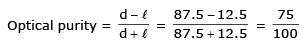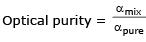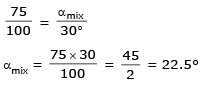QUESTION: 2

### When an optically active compound is placed in a 10 dm tube is present 20 gm in a 200 ml solution rotates the PPL by 30º. Calculate the angle of rotation & specific angle of rotation if above solution diluted to 1 Litre.

Solution: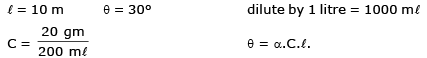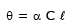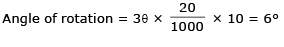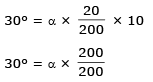specific angle of rotation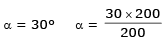QUESTION: 3

### In the given following compound find out the pair of enantiomers and diastereomers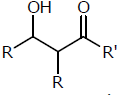Solution: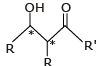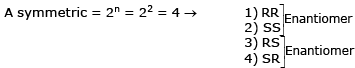1,3    1,4    2,3    2,4 are Diastereomers.
Ans. 2,4

QUESTION: 4

The molecule (s) that exist as meso structure(s)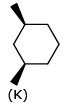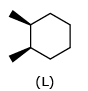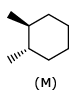Solution: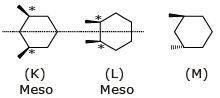Both K and L meso structure.

QUESTION: 5

Among the following, a pair of resolvable configurational enantiomers is given by

Solution: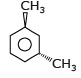Plane not present so optically active ⇒ Resolvable
Trans-1,3-dimethyl cyclo hexane.

QUESTION: 6

Which two of the following compounds are identical ?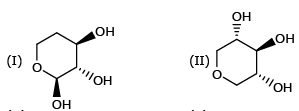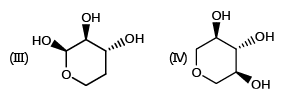Solution: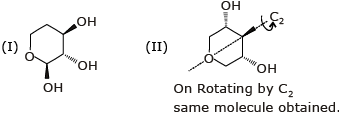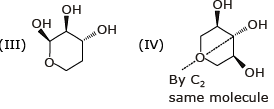Identicle compounds are = II & IV

QUESTION: 7

Which two of the following compounds are diasteromers ?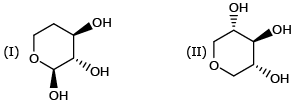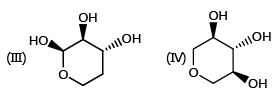Solution:I & III are diastereomers because they are not mirror image.

QUESTION: 8

Which of the following is properly classified as a meso compound ?

Solution: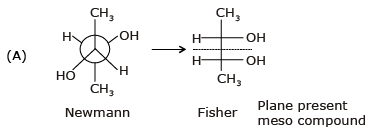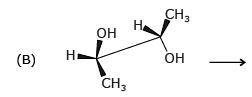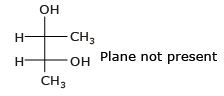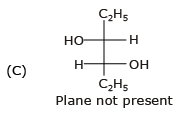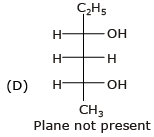So compound (A) is meso compound

QUESTION: 9

Which two of the following compounds represents a pair of enantiomers ?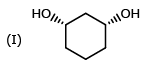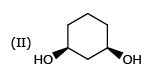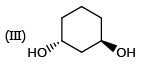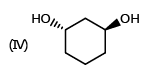Solution: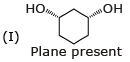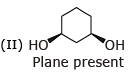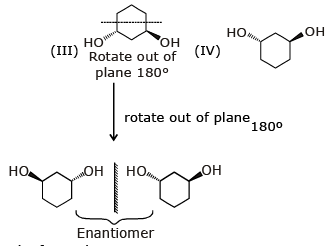III and IV compound represents pair of enantiomers.

QUESTION: 10

The drawing on the rigth shows that trans-1, 3-dichlorocyclohexane is chiral. Efforts to resolve this compound fail. Why ?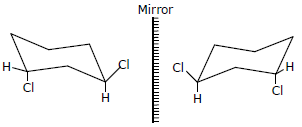Solution:

They are conformers as well as enantiomers.

QUESTION: 11

How many stereoisomers of (CH3)2CHCH = CHCH2CH(OH)CH2Br are possible ?

Solution: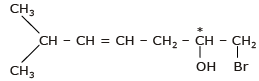asymmetric T.S.I = 2n = 22 = 4
n = no. of double bond + no. of chiral center = 1 + 1 = 2

QUESTION: 12

What common symmetry of elements if any are found in the stable chair conformer of trans-1, 2- dichlorocyclohexane ?

Solution: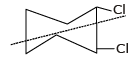A single C2 rotational axis but no mirror plane.

QUESTION: 13

How many stereoisomer are possible for the following molecule ?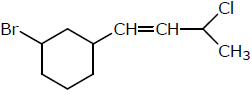Solution: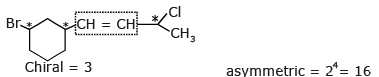double Bond for G.I. = 1
n = 3 + 1 = 4

QUESTION: 14

How many stereoisomers are possible for the following compound ?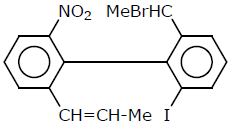Solution: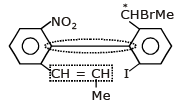Asymmetric 23 = 8

QUESTION: 15

Optical rotation produced by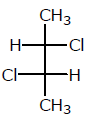is 36° then that product by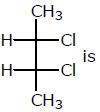Solution:

Ind compound is meso compound so θ = 0°

QUESTION: 16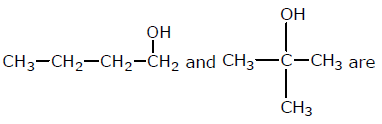Solution: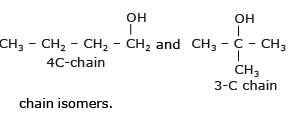QUESTION: 17

Dextrorotatory α-pinene has a specific rotaiton [α]D20 = +51.3°. A sample of α-pinene containing both the enantiomers was found to have a specific rotationa value [α]D20 = +30.8°. The percentages of the (+) and (–) enantiomers present in the sample are, respectively.

Solution: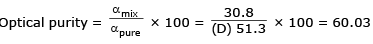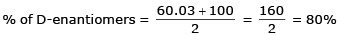% of L-enantiomer = 100 – 80 = 20%

QUESTION: 18

(+)-mandelic acid has a specific rotation of 158°. What would be the observed specific rotation of a mixture of 25% (–)-mandelic acid and 75% (+)-mandelic acid ?

Solution: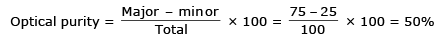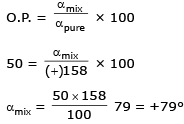QUESTION: 19

Number of structural isomers of compound having molecular formula C4H7Cl.

Solution: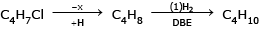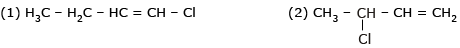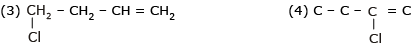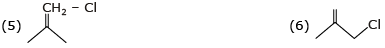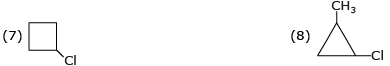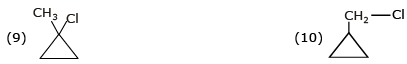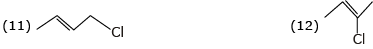Total 12 structure isomer possible

QUESTION: 20

Which of the following sugars has the configuration (2S 3R, 4R) ?

Solution: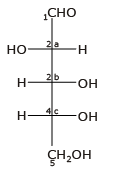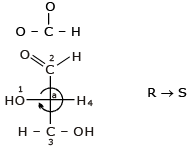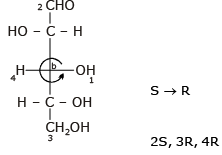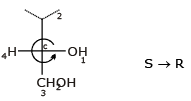QUESTION: 21

Which of the following statements must be true for two pure chiral isomers ?

Solution:

Two pure chiral center must be optically active.

QUESTION: 22

Which of the following statements is true for a pair of diastereomers ?

Solution:

Physical properties like M.P., B.P., solubility are different for diastereomers.

QUESTION: 23

How many stereoisomer may have this natural occuring compound.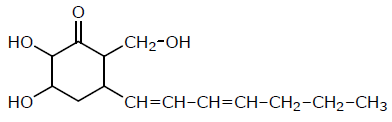Solution: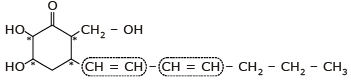n = 6 asymmetric = 26 = 64

QUESTION: 24

An optically pure compoud X gave an [α]D25 = +20.0°. A mixture of X and its enantiomer Y gave [α]D25 = +10°. The ratio of X to Y in the mixture is

Solution: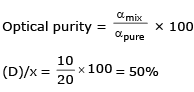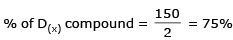(y) compound = 100 – 75 = 25%
(x) : (y)
75 : 25
3 : 1

QUESTION: 25

Molecular formular C3H6Br2 can have (including stereoisomers):

Solution:

C3H6Br2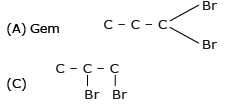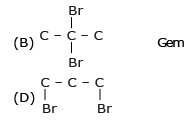Two gem dibromide.